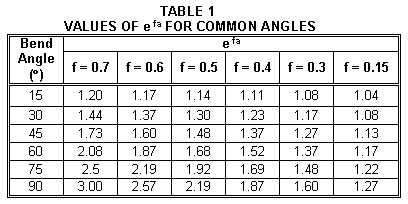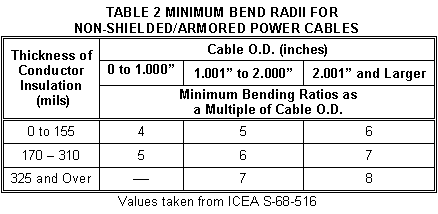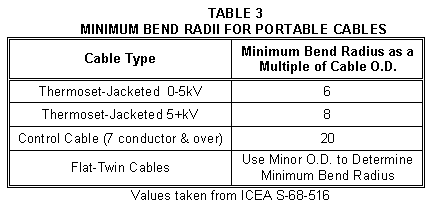NEWS

Henan Xinchang Import and Export Trading Co.,Ltd

power cable pulling tension

26/03/2019

dear friends, when cable is installed, why you should know its pulling tension at first?

is it a necessary data when cable installed, here the answer is yes.

let us learn how to have cable pulling tension from the following , thanks.

The following data is intended as a general guide to aid individuals with the installation of cables inside ducts, raceways or conduit. These recommendations are based upon a study sponsored by the ICEA (Insulated Cable Engineers Association). The information contained herein is not a comprehensive set of instructions. An engineer or technician experienced with installations should be consulted for particular applications where out-of-the-ordinary conditions exist or could be encountered.

Preface
Before installing cables, it is recommended that the routing be thoroughly inspected to avoid bends and/or pulling tensions that exceed specified limits. Good design of the duct, raceway or conduit itself along with the routing is essential to ensure damage-free installation and the full service life of all components.

Maximum pulling tension on a cable
For cable equipped with pulling eye or pulling bolt, the formula shown below is used to calculate the maximum allowable pulling tension on the cable for the entire routing.

Tm = K x n x CMA (Formula 1)
Where:Tm = maximum pulling tension (lbs.) (see Appendix A for calculated tensions)
K = constant
• 0.008 for copper conductors
• 0.006 for aluminum conductors
n = number of conductors
CMA = circular mil area for one conductor

Maximum permissible pulling length
The maximum length of cable that can safely be pulled through conduit is calculated as shown below.

Lm = Tm / ( W x f ) (Formula 2)
Where:Lm = maximum pulling length, feet
Tm = maximum pulling tension, lbs.
W = weight of cable, lbs./ft.
f = coefficient of friction (if unknown, use 0.5)

Cable grip tension
When cable grip is used over non lead-jacketed cable, the pulling tension should not exceed 1,000 lbs. or 1,000 lbs. per grip (when used with multi-conductor cables) and the tension calculated in Formula 1.

Calculated pulling tension – straight section of conduit

Ts = L x W x f (Formula 3)
Where:Ts = pulling tension at end of straight section, lbs.
L = length of straight section, feet
W = weight of cable, lbs./ft.
f = coefficient of friction (if unknown, use 0.5)

Calculated pulling tension – curved (bent) section of conduit

Tb = Ts x efa (Formula 4)
Where:Tb = pulling tension at end of bend, lbs.
Ts = pulling tension at end of straight section entering the bend, lbs.
e = naperian log base (2.718)
f = coefficient of friction (if unknown, use 0.5)
a = angle of bend (radians)
(See Table 1 with efa values for common angles and Tables 2 & 3 for minimum bend radii)

Maximum sidewall pressure at bends
Sidewall pressure is caused by the tension in the cable acting horizontally and the weight of the cable acting vertically. Generally, the tension of a cable immediately as it leaves a bend must not be greater than 300 times the bend radius (in feet), and the maximum sidewall pressure must not exceed 300 lbs./ft. Shown below are formulas to calculate the maximum allowable tension at a bend and the actual sidewall pressure.

Tbm = 300 x r (Formula 5)
Where:Tbm = maximum allowable pulling tension at bend, lbs.

P = Tb / r (Formula 6)
Where:P = actual sidewall pressure on cable, lbs./ft.
Tb = pulling tension at and of bend, lbs.

The maximum allowable pulling tension at bend (Tbm) is the limit that the calculated pulling tension (Tb) should be compared. If Tb is greater than Tbm, the possibility of redesign or re-routing should be considered.Example – 1/0 AWG THHN, cable weight = 0.37 lbs./ft.MAXIMUM PULLING TENSION, Tm

Tm = .008 x 1 x 105600 = 846 lbs.

MAXIMUM PERMISSIBLE PULLING LENGTH, Lm

Lm = 845 / (.37 x 0.5) = 4,568 ft

PULLING FROM POINT "A"

• Tension @ A = Zero

• Tension @ B (Ts1) = 200 x .37 x .5 = 37 lbs.

• Tension @ C (Tb1) = 37 x 1.48 = 55 lbs. [ PB-C = 55 / 10 = 5.5 lbs./ft ]

• Tension @ D (Ts2) = 55 + [70 x .37 x .5] = 68 lbs.

• Tension @ E (Tb2) = 68 x 2.19 = 149 lbs. [ PD-E = 149 / 10 = 14.9 lbs./ft ]

• Tension @ F (Ts3) = 149 + [100 x .37 x .5] = 168 lbs.

• Tension @ G (Tb3) = 168 x 1.48 = 249 lbs. [ PF-G = 249 / 10 = 24.9 lbs./ft ]

Tension @ H (Ts4) = 249 + [50 x .37 x .5] = 258 lbs.

PULLING FROM POINT "H"

• Tension @ H = Zero

• Tension @ G (Ts1) = 50 x .37 x .5 = 9 lbs.

• Tension @ F (Tb1) = 9 x 1.48 = 13 lbs. [ PG-H = 13 / 10 = 1.3 lbs./ft ]

• Tension @ E (Ts2) = 13 + [100 x .37 x .5] = 32 lbs.

• Tension @ D (Tb2) = 32 x 2.19 = 70 lbs. [ PE-D = 70 / 10 = 7 lbs./ft ]

• Tension @ C (Ts3) = 70 + [70 x .37 x .5] = 83 lbs.

• Tension @ B (Tb3) = 83 x 1.48 = 123 lbs. [ PC-B = 123 / 10 = 12.3 lbs./ft ]

Tension @ A (Ts4) = 123 + [200 x .37 x .5] = 160 lbs.

Pulling from either direction will not result in the tension or sidewall pressure exceeding the limits (846 lbs. & 300 lbs./ft). However, pulling from point "H" will result in approximately 40% less tension being placed on the cable. Therefore, it is most desirable to pull from point "H".

General guidelines for cable pulling
PREPARATION:

1. Establish the direction of the pull based on safe pulling tensions and sidewall pressure calculations.
2. Select the correct size pulling eyes, bolts or grips.
3. Locate feeder reels, spools, etc. such that the tension at the feeding end is minimized.
4. Use pulling equipment that provides smooth speed control.
5. Choose a pulling rope that has the required tensile strength.
6. Before pulling, make sure the conduit is clean and free of dirt, water, scale, etc.
7. For long and/or heavy pulls, pre-lubricate the conduit and pull rope, particularly when using PVC.
8. Install a dynamometer.

CABLE PULLING:

1. Apply pulling lubricant (compound) liberally during the installation.
2. If possible, use two-way communication at both ends of the run, particularly on long runs.
3. Accelerate slowly and smoothly to a constant pulling speed.
4. Avoid letting the cable stop part way through the pull. The friction is greatly increased when re-starting a pull.

AFTER PULLING:

1. Seal the ends of the installed cable to prevent moisture from getting inside the cable.
2. High voltage testing is recommended before and after the installation.the above is a knowledge for cable pulling tension, share with it on our internet, in good hope to help someone who are working on cable industry, and  our factory are glad to work together with you on power cable, control cable, overhead distribution lines cable and conductor, and underground cables. good price and best quality would be double yours business work, waiting for yours kind inquiry.